# Programming Videos

## Search

### Binary Search Program in C, C++

Write a C, C++ program to implement a binary search. Binary search is an efficient search algorithm as compared to linear search.  Let's implement this algorithm in  C, C++.

## Binary Search

Binary search is a search algorithm that finds the position of an element (target value) within a sorted array. It is also known as half-interval search or logarithmic search.

Important Points

i) Always use binary search with sorted values (either in asc or desc order).

Bubble sort algorithm and their implementation

Quicksort algorithm and their implementation

ii) Time complexity of binary search.

Best case performance - O(1)

Average and worst case time complexity - O(logn)

Binary Search using Recursion in C, C++

Sorting algorithm and their time complexity

## Binary Search Algorithm

```    low = 0;
high = size - 1;

while (low <= high) {

/* Calculate mid index */

mid = (low + high) / 2;

/* If element is found at mid index
then return the index */

if (arr[mid] == num ) {

return mid+1;

} else if ( arr[mid] > num) {

high = mid - 1;

} else if ( arr[mid] < num) {

low = mid + 1;

}
}

return -1;
```

## Binary Search Program in C++

```#include<iostream.h>
#include<conio.h>

void main()
{

int arr,beg,mid,end,i,n,num;

cout << "\n Enter the size of an array ";
cin >> n;

cout << "\n Enter the values in sorted order (asc or desc) \n";

for(i = 0; i < n;i++)
{
cin >> arr[i];
}

/* Initialize beg and end value. */

beg = 0;
end = n-1;

cout << "\n Enter a value to be searched in an array ";
cin >> num;

/* Run loop, while beg is less than end. */

while( beg <= end)
{

/* Calculate mid. */

mid = (beg+end)/2;

/* If value is found at mid index,
the print the position and exit. */

if(arr[mid] == num)
{
cout << "\nItem found at position "<< (mid+1);

exit(0);

} else if(num > arr[mid]) {

beg=mid+1;

} else if (num < arr[mid]) {

end=mid-1;

}

}

}
```

Output:

Enter the size of an array : 7

Enter the values in sorted order (asc or desc) :

3    5    6    8   9   12  13

Enter a value to be search :  6

Item found at position : 3

## Binary Search Program in C

```#include <stdio.h>

/* Search number in an array using binary search algorithm */

int binarySearch (int arr[], int num, int size) {

int low, high, mid;

low = 0;
high = size - 1;

while (low <= high) {

/* Calculate mid index */

mid = (low + high) / 2;

/* If element is found at mid index
then return the index */

if (arr[mid] == num ) {

return mid+1;

} else if ( arr[mid] > num) {

high = mid - 1;

} else if ( arr[mid] < num) {

low = mid + 1;

}
}

return -1;

}

int main(void) {

int arr, len, num, result;

printf(" \n Enter the size of an array (Max 100)");
scanf ("%d", &len);

printf("\n Enter the elements of an array in asc or desc order");

/* Taking array input values from user */

for (int i = 0; i < len; i++) {

scanf ("%d", &arr[i]);

}

printf ("\n Enter the elements to be searched in an array");
scanf ("%d", &num);

result = binarySearch (arr, num, len);

if (result == -1) {

} else {

printf (" \n Number is found at %d position", result );
}

return 0;
}
```

Output :

Enter the size of an array (Max 100) : 5

Enter the elements of an array in asc or desc order : 2   3    6   7   8

Enter the elements to be searched in an array : 7

Number is found at 4 position

Programming questions on Strings

Programming questions on Recursion

Programming questions on Array

1.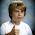#include
for exit(0) function

1.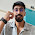2.2.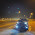1.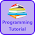I am glad that this code helps you.

3.i only understand binary search from this

4.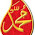I understand binary search only from this

5.What is the worst case of binary search Algorithm?

1.Worst case of binary search occur when element you are searching is not present in array or list.

6.helps me

1.What kind of help do you need in Binary Search program.

7.what is the expected output ?

1.What's the input you are entering.

8.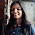is 'loc' of the input value not required to find the position ?

9.10.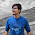I couldn't figure out the last few lines of the code, pls help me

11.I couldn't figure out the logic of what happens if num>Arr[mid] pls help me!!

1.If num > arr[mid] then set beg flag to mid + 1 as number is greater than mid so you will search the item in second halve.

12.No, choice of variable is our choice, we can choose any name of the variable

13.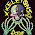Use stdlib.h for exit 0 function

14.Use stdlib.h for exit 0 function

15.What about dealing with string arrays or char arrays??

16.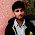this binary search code is wrong
you can't write mid=(low+high)/2 . It will create overflow.Assume that you have an array of size 2^32 and low = 2^32-2 and high = 2^32-1 so low +high will create overflow .
So the correct code will be mid = low+(high-low)/2;

1.17.Thanks man, it was great help!!

18.what if we have two same numbers at different positions in an array and we want to find the position of both using BS??????

19.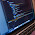a program that input a ten number by user and search a number given by user by using binary search.

20.write a program that input a number by user and search number given by user by using binary search .

1.#include
using namespace std;
main()
{
int beg,mid,end,i,n,num;
int a={4,5,6,7,8,9,10,11,14,15};
//initialize the beg & end value
beg=0;
end=10-1;
cout<<"/n entr the value to search :";
cin>>num;
//run loop when bega[mid])
{
beg=mid+1;
}
else if(num<a[mid])
{
end=mid-1;
}
else
}

}

21.Thanks for helping last cout<<"number not found" became before the close bracket in this way:
end=mid-1;
}
else
}
}
Binarey search in C++

22.nice solution

23.In the case of beg>end the ouptput is not found number in list etc...but in same case is it possible to use the do...while loop or switch case for change the value of *end* for take some value in output..?
If is it possible plzz give code for it.

24.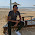1-i dont understand why you made while loop low<=end
2-why you made equale in while loop
3- what is the exit(0)? what does it make ?!

25.1- i didn't understand why you made while loop low<=high ?
2- why you made equale in while loop?
3- what is the function exit(0)? what does it mean and what can i do by using it ?

26.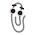Does not work "at all" in descending order.